# Using Many Models to Compare Datasets

#### 2023-07-24

The problem of comparing datasets arises frequently, and this note describes a simple strategy that converts this comparison into a binary classification problem. The basic idea is to merge the datasets, define a binary source indicator that identifies the original dataset from which each record was taken, and fit a binary classifier that predicts this source indicator. The datarobot R package is used here to invoke the DataRobot modeling engine, which builds a collection of different classifiers from this merged dataset, allowing us to compare results across many models. In particular, classifier quality measures like area under the ROC curve (AUC) can be used to assess the degree of difference between the original datasets: small AUC values suggest that the original datasets are similar, while large AUC values suggest substantial differences. If differences are detected, the random permutation strategy described in the companion vignette “Assessing Variable Importance for Predictive Models of Arbitrary Type” can be applied to determine which variables from the original datasets are most responsible for their differences. These ideas are illustrated here with two examples. The first considers the question of whether missing serum insulin values from the Pima Indians diabetes dataset from the mlbench package - coded as zeros - appear to be systematic, while the second examines a subset of anomalous loss records in a publicly available vehicle insurance dataset.

## 1. Introduction

The problem of comparing datasets (or subsets of a given dataset) is an important one in a number of applications, e.g.:

1. A predictive model now in use was developed from historical customer data: has customer behavior changed enough with time that the model should be rebuilt?
2. A dataset has a significant fraction of missing values for key variables (e.g., the response variable or key covariates that are believed to be highly predictive): does this missing data appear to be systematic, or can it be treated as random?
3. An unusual subset of records has been identified (e.g., based on their response values or other important characteristics): is this subset anomalous with respect to other variables in the dataset?

In all of these cases, there are two questions of interest: first, is there evidence of a difference between these datasets, and second, if so, which variables are responsible for these differences?

This vignette describes and illustrates a simple strategy for reformulating these questions in terms of a binary classification problem: merge the datasets, define a binary source indicator, and build a collection of classifiers that predict this source indicator from other variables in the combined dataset. If the datasets are substantially different, it should be possible to accurately predict the source indicator from these other variables. Thus, traditional measures of classifier performance (e.g., area under the ROC curve) can be used to assess the degree of difference between the datasets, and permutation-based variable importance measures can be used to identify those variables most responsible for these differences. More specifically, this vignette describes the use of the datarobot R package to implement the strategy just described, taking advantage of the fact that the DataRobot modeling engine builds a collection of different classifiers, all based on the same modeling dataset.

Two examples are presented. The first considers the question of whether missing insulin values from the PimaIndiansDiabetes dataframe in the mlbench R package appear to be systematic or random. Specifically, almost half of the observed values for this variable are recorded as zero, a physically impossible value apparently used to code missing data: the question considered here is whether these records appear to differ systematically with respect to the other variables in the dataset. The second example considers an anomaly that appears in the nonzero loss records from an Australian vehicle insurance dataset. As is typical of policy-level insurance datasets, the overwhelming majority of these losses are zero, but the smallest nonzero loss value observed is exactly AU$200, representing approximately 15% of all nonzero losses. The question considered here is whether these unusual “low loss” records differ systematically from the other, more typical nonzero loss records in the dataset. ## 2. Example 1: missing data Missing data is a problem that arises frequently and has been widely discussed in the statistics literature (see Little and Rubin (2002) for a useful overview). Often, missing data observations are assumed to be missing completely at random (MCAR), meaning the probability that the $$k^{th}$$ observation $$x_{ik}$$ of the variable $$x_i$$ is missing does not depend on either observed or unobserved data values. Under this assumption, missing data reduces the effective sample size, increasing the variability of estimates computed from the data, but it does not introduce biases. In contrast, systematic missing data can introduce biases that, in unfavorable cases, can be severe. In general, systematic missing data can be difficult to detect: if, for example, large values of $$x_{ik}$$ are more likely to be missing than small values, independent of all other variables in the dataset, this may not be detectable from the available data. In other cases, termed missing at random (MAR), systematically missing data records may differ for different values of other variables in the dataset: e.g., the probability that $$x_{ik}$$ is missing may depend on the value of other variables $$x_{jk}$$ that are observed. In these cases, we may be able to learn something useful by comparing records with missing $$x_i$$ values with those with non-missing $$x_i$$ values. The following example illustrates this idea. ### 2.1 The Pima Indians diabetes dataset The Pima Indians diabetes dataset is available from the University of California at Irvine Machine Learning Repository, and different versions have been included in several R packages. The version used here is from the mlbench package (Leisch and Dimitriadou 2010), and it is identical to that available from the UCI repository; other versions differ, particularly in their treatment of missing data. This dataset describes 768 female members of the Pima Indians tribe; R’s built-in str function gives this summary: library(mlbench) data(PimaIndiansDiabetes) str(PimaIndiansDiabetes) ## 'data.frame': 768 obs. of 9 variables: ##$ pregnant: num  6 1 8 1 0 5 3 10 2 8 ...
##  $glucose : num 148 85 183 89 137 116 78 115 197 125 ... ##$ pressure: num  72 66 64 66 40 74 50 0 70 96 ...
##  $triceps : num 35 29 0 23 35 0 32 0 45 0 ... ##$ insulin : num  0 0 0 94 168 0 88 0 543 0 ...
##  $mass : num 33.6 26.6 23.3 28.1 43.1 25.6 31 35.3 30.5 0 ... ##$ pedigree: num  0.627 0.351 0.672 0.167 2.288 ...
##  $age : num 50 31 32 21 33 30 26 29 53 54 ... ##$ diabetes: Factor w/ 2 levels "neg","pos": 2 1 2 1 2 1 2 1 2 2 ...

An important aspect of this dataset is summarized in the following note, from the metadata included with the UCI posting:

UPDATE: Until 02/28/2011 this web page indicated that there were no missing values in the dataset. As pointed out by a repository user, this cannot be true: there are zeros in places where they are biologically impossible, such as the blood pressure attribute. It seems very likely that zero values encode missing data. However, since the dataset donors made no such statement we encourage you to use your best judgement and state your assumptions.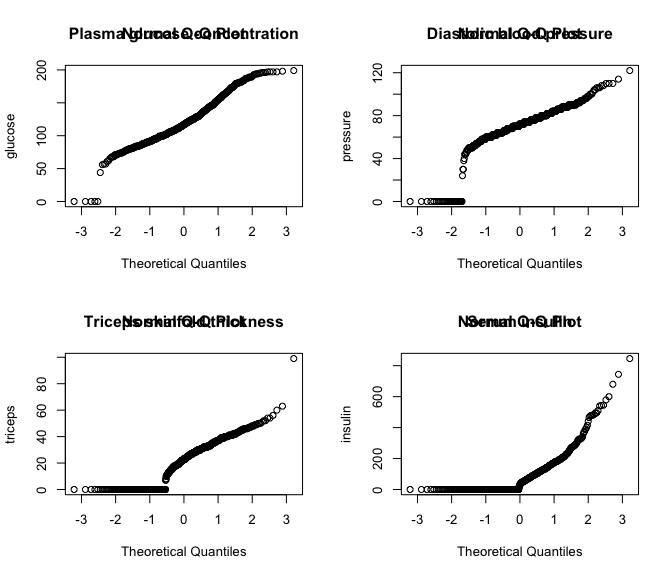Figure 1: Normal QQ plots of four Pima Indians diabetes variables.

Figure 1 shows normal quantile-quantile plots for four of the variables in the dataset, generated using the qqnorm function. (Fox and Weisberg 2011): plasma glucose concentration (upper left), diastolic blood pressure (upper right), triceps skinfold thickness (lower left), and serum insulin (lower right). These plots all exhibit an exaggerated lower tails, each representing repeated zeros in the data. The most extreme case is that of insulin, where 48.7% of the recorded values are zero. This note assumes these zeros represent missing data, and the question considered here is whether these missing values are systematic.

### 2.2 Is insulin systematically missing?

To apply the approach proposed here to the missing insulin data from the Pima Indians diabetes dataset, first create a binary response variable insulinMissing that is equal to 1 if insulin is missing (i.e., zero), and 0 otherwise. Next, remove insulin from the original dataset - since it is a postdictor of our response variable (i.e., a perfect predictor, using inadmissable information) - and replace it with insulinMissing:

insulinMissing <- as.numeric(PimaIndiansDiabetes$insulin == 0) modifiedPima <- PimaIndiansDiabetes modifiedPima$insulin <- NULL
modifiedPima$insulinMissing <- insulinMissing This modified dataset is then used to set up a DataRobot modeling project that builds models to predict the response variable insulinMissing. As described in the companion vignette “Introduction to the DataRobot R Package,” this is a two-step process. First, the data source is uploaded and modeling is started with the StartProject function: insulinProject <- StartProject(dataSource = modifiedPima, projectName = "InsulinProject", target = "insulinMissing", wait = TRUE) Since no fitting metric is specified explicitly in this function call, the DataRobot default is used, which is LogLoss for this example. Once the model-fitting is complete, a detailed summary of the project models, their associated preprocessing, and their performance by various measures can be obtained with the ListModels function. insulinModelList <- ListModels(insulinProject)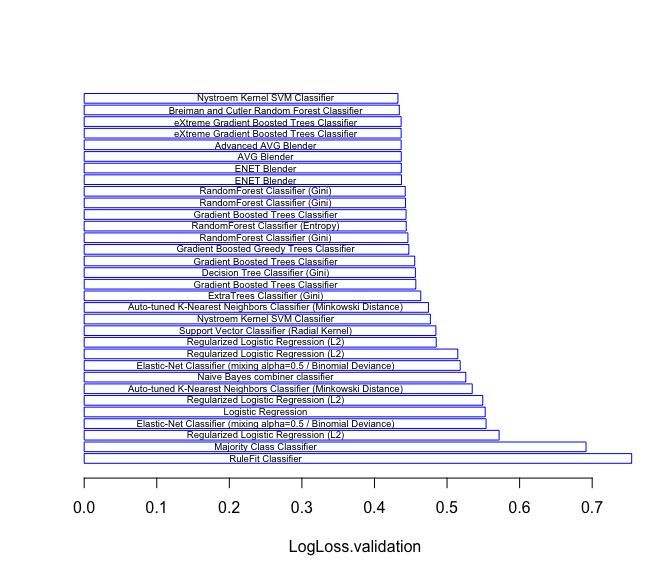Figure 2: Barplot of LogLoss values for the models predicting missingInsulin. A horizontal barplot summary of the classifier types and their LogLoss performance is shown in Figure 2, generated by the plot method for the S3 object of class “listOfModels” returned by ListModels. Of the 32 models in this project, the one with the best (i.e., smallest) LogLoss value is: ##  "Nystroem Kernel SVM Classifier::Regularized Linear Model Preprocessing v4" This model appears at the top of this plot, while the model RuleFit Classifier::One-Hot Encoding::Missing Values Imputed shows the worst performance and appears at the bottom of the plot. Interestingly, this model exhibits even worse performance than the Majority Class Classifier, a trivial model that assigns probability 1 to the most frequently occurring class (“insulin not missing”) for all data records, included as a performance benchmark for all of the other models. The fact that all other models exhibit substantially smaller LogLoss values suggests that the missing insulin values are at least somewhat predictable from the other covariates in the dataset. A clearer view of the predictability of the missing insulin values may be seen in Figure 3, which shows the area under the ROC curve (AUC) computed for each model, one of the response variables included in the model summary information returned by the ListModels function. The only model with an AUC value substantially less than 0.75 is the trivial majority rule classifier, which achieves an AUC value of 0.50, essentially equivalent to random guessing. The best model is marked with a red dot in this plot, and it achieves an AUC value of 0.818, suggesting that the missing insulin values are strongly predictable from the values of the other covariates. In fact, the AUC value exceeds 0.80 for 59.4% of the models in this project, further supporting this conclusion.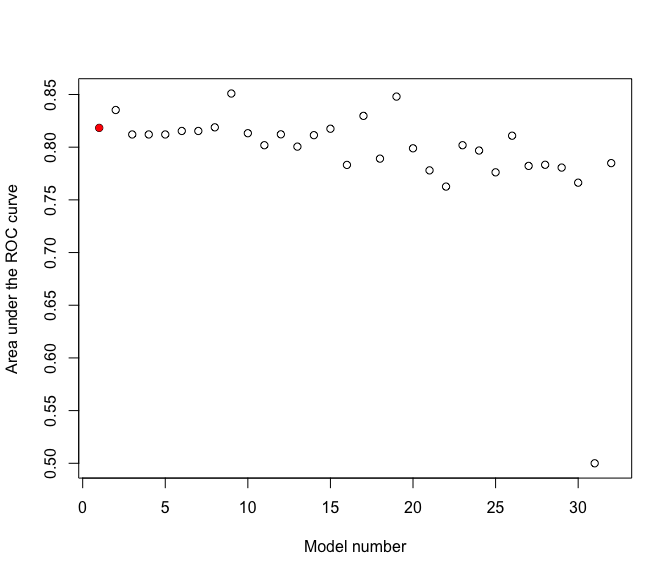Figure 3: Plot of AUC values for the models predicting missingInsulin. ### 2.3 Assessing variable importance Given the evidence just presented for a systematic difference between the Pima Indians diabetes records with missing insulin values and those without, the next question of interest is what we can say about the nature of these differences. For example, if patients who have been diagnosed as diabetic are much more (or less) likely to have missing insulin values than those diagnosed as non-diabetic, the issue of treating these missing data values becomes especially important in avoiding biases in models developed to predict this diagnosis. In particular, simply omitting these records would be a poor strategy, as it could lead to strongly biased predictions. To address this question - i.e., “what is the nature of these differences?” - the following paragraphs adopt the random permutation strategy for assessing variable importance described in the companion vignette, “Assessing Variable Importance for Predictive Models of Arbitrary Type.” Briefly, the idea is to apply a random permutation to each variable used to predict insulinMissing, create a new modeling project based on this modified covariate, and compare the performance achieved using the randomized covariate with the original performance. If we apply this randomization strategy to a variable that is highly predictive of the response, the quality of the project models should suffer significantly, while if we apply this strategy to a non-predictive covariate, we should see little or no effect. To implement this approach, the function PermuteColumn described in the companion vignette is used to create a modified dataset with a random permutation applied to one of the covariates. Then, the function StartProject is used to upload this modified dataset and starts the model-building process. The model-fitting results returned by the ListModels function after the project has completed are then saved and used to compute the permutation-induced shifts in the fitting metric that provide the basis for assessing variable importance. More specifically, the following R code constructs a list containing the model information from the original data (in the first list element), followed by the results obtained by applying the same random permutation to each of the eight covariates in the modifiedPima dataset: modelList <- list(n = 9) modelList[] <- insulinModelList allVars <- colnames(modifiedPima)[1:8] permFile <- tempfile(fileext = "permFile.csv") for (i in 1:8) { varName <- allVars[i] PermuteColumn("modifiedPima.csv", varName, permFile) projName <- paste("PermProject",varName,sep="") permProject <- StartProject(permFile, projectName = projName, target = "insulinMissing", wait = TRUE) modelList[[i+1]] <- ListModels(permProject) } The list returned by this function forms the input for the function PermutationMerge described in the variable importance vignette, which constructs a dataframe containing the original and modified fitting metric values for each project model (as defined by unique blueprintId values), along with key model information. This dataframe, in turn, can be used with the function ComputeDeltas, also described in the variable importance vignette, to construct a dataframe containing the original fitting metric values and the permutation-induced differences. The results obtained by applying the functions PermutationMerge and ComputeDeltas to the list returned by the code listed above form the basis for Figure 4.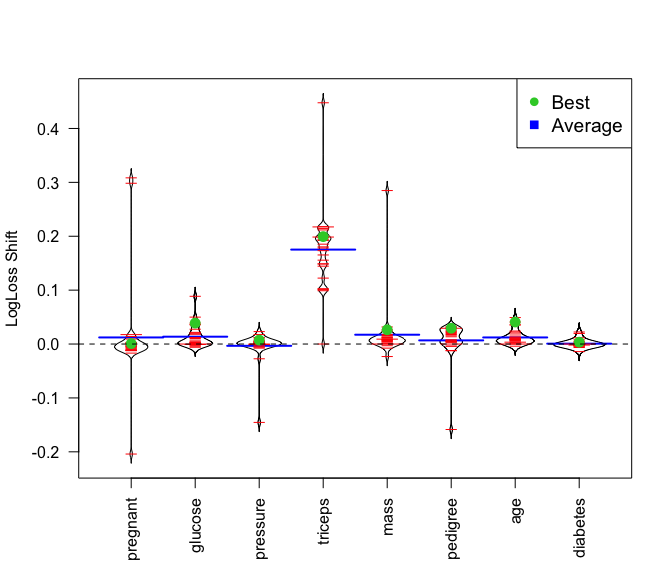Figure 4: Beanplot summary of LogLoss shifts versus random permutation. This figure is a collection of beanplots (Kampstra 2008), each summarizing the change in LogLoss value that results when a random permutation is applied to the indicated covariate. In each plot, individual models are represented by the short red lines, and the average LogLoss shift for all models is indicated by the wider blue line. Also, the LogLoss shifts for the best model - here, a Nystroem kernel support vector machine classifier - are indicated by green dots in each beanplot. It is clear from these results that triceps skinfold thickness is the most influential variable for almost all models. There are, however, a few outlying models that exhibit extreme dependencies on certain variables that are not generally influential. For example, the very large increase in LogLoss seen when the random permutation is applied to the pregnant variable corresponds to two models: an auto-tuned K-nearest neighbor classifier based on the Minkowski distance, and a decision tree classifier based on the Gini norm, which also exhibits the strongest dependence on triceps of any model. Interestingly, the extreme dependence seen on mass for one case corresponds to the same auto-tuned K-nearest neighbor classifier, but with different preprocessing applied. The influence of the triceps variable can also be assessed by looking at the individual AUC values for the original models and those with the random permutation applied to triceps. These values are simply generated, again using the MergePermutations function, but specifying metric as the non-default value AUC.validation. The results are shown Figure 5, which compares the original AUC values (open circles) with those for the same models fit to the randomized triceps variable (solid red triangles). It is clear from these plots that removing the triceps variable from almost any of these models causes a substantial reduction in their ability to predict the missing insulin indicator. The sole exception is the trivial majority class classifier, which has no real predictive power and does not depend on any covariates, so its AUC value is 0.50 either with or without the triceps variable.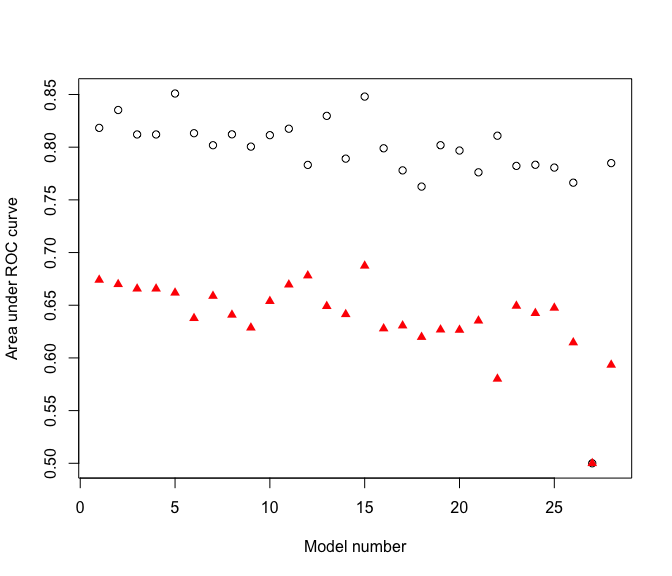Figure 5: Plot of AUC values for the original models (open circles) and those for the models with the random permutation applied to triceps (solid red triangles) The strong influence seen for the triceps skinfold thickness in predicting missing insulin values for this example raises the obvious question, “Why?” Recall from the plots in Figure 1 that triceps also exhibited a large fraction of missing data values, coded as zero. One possibility, then, is that these missing values are strongly associated. In fact, this appears to be the case, as evident from the following contingency table of missing values for insulin and triceps: ## missingTriceps ## missingInsulin 0 1 ## 0 394 0 ## 1 147 227 In particular, note that triceps is never missing when insulin is present, and it is about 50% more likely to be missing than present when insulin is missing. While this observation does not give a complete explanation for why insulin is missing in this dataset, it does show that missing values in these two variables are strongly linked, suggesting a common mechanism for their absence. As a practical matter, this means that any missing data treatment strategy adopted in analyzing this dataset needs to account for this relationship. ## 3. Example 2: data anomaly characterization Sometimes, a non-negligible subset of records in a dataset exhibits an unusual characteristic (e.g., an anomalous response value). In such cases, it may be of considerable interest to understand whether these records also differ systematically with respect to other characteristics, and, if so, what these differences are. The following sections describe a specific example. ### 3.1 An Australian vehicle insurance dataset The following example is based on an Australian vehicle insurance dataset, available from the website associated with the book by deJong and Heller (2008) and also from the insuranceData R package: library(insuranceData) data(dataCar) This dataset characterizes 67856 single-vehicle, single-driver insurance policies, giving values for 11 variables, none of which appear to exhibit missing data values. There are three possible response variables in this dataset: (1), the binary claim indicator clm; (2), the claim count numclaims (which varies from 2 to 4 in this dataset for the rare cases where the policy files multiple claims); and (3), the loss associated with the claim(s), claimcst0. As is typical for policy-level insurance data, only a small fraction of policies file claims, and this example considers only this subset of records: lossIndex <- which(dataCar$claimcst0 > 0)
keepVars <- c("veh_value", "exposure", "claimcst0", "veh_body", "veh_age",
"gender", "area", "agecat")
lossFrame <- subset(dataCar, claimcst0 > 0, select = keepVars)

Since the following variables are irrelevant to the question considered here, they were omitted to simplify subsequent analysis:

1. the binary claim indicator clm has the value 1 for all of the nonzero loss records;
2. the claim count variable numclaims is nonzero if and only if claimcst0 is nonzero;
3. the variable X_OBSTAT_ has only one value for all records in the dataset.

{r echo = FALSE, fig.width = 7, fig.height = 6, fig.cap="Figure 6: Two views of the log of the nonzero loss values.", warning = FALSE, message = FALSE} layoutMatrix <- matrix(c(1, 0, 0, 2), nrow = 2) layout(layoutMatrix) plot(density(log(lossFrame$claimcst0)), main = "Log nonzero loss, \n estimated density") qqPlot(log(lossFrame$claimcst0), ylab = "Log nonzero loss value") title("Normal Q-Q plot") arrows(-2, 7.5, -2, 6) text(-2, 8, "$200 exactly") A nonparametric density estimate for the log of the nonzero loss values is shown in the upper plot in Figure 6, where the log transformation has been applied because these loss values span nearly three orders of magnitude. The key feature seen in this plot is the multimodal character of the density, suggestive of distributional heterogeneity. Such heterogeneity frequently arises in insurance data when losses represent aggregates from multiple sources (e.g., comprehensive automobile insurance policies that cover both relatively small losses like broken windshields and large losses like car theft). Here, however, the left-most peak in this density arises from an unusual subset of the loss data records. The existence of this anomalous data subset is highlighted by the normal QQ plot shown in the lower plot in Figure 6. In particular, note the flat lower tail in this plot, similar to those caused by the zeros in the Pima Indians diabetes dataset. Here, however, this lower tail corresponds to the value of$200 exactly, it represents the smallest nonzero value for claimcst0 in this dataset, and it corresponds to approximately 15% of the total nonzero loss records. The question considered here is whether this unusual subset of “small losses” differs systematically from the other nonzero loss records with respect to the other covariates in the dataset, and if so, how?

### 3.2 Characterizing the “small loss” records

To characterize this “small loss” subset, proceed as before, first replacing the claimcst0 value in the original dataset with the binary indicator anomalousLoss, taking the value 1 if claimcst0 is equal to $200, 0 if claimcst0 has some other nonzero value, and excluding all other records. Next, set up a DataRobot modeling project to build a collection of binary classifiers, and finally retrieve the modeling results with ListModels: anomaly <- as.numeric(lossFrame$claimcst0 == 200)
anomFrame <- lossFrame
anomFrame$claimcst0 <- NULL anomFrame$anomaly <- anomaly
anomProject <- StartProject(anomFrame, projectName = "AnomalyProject", target = anomaly, wait = TRUE)
anomalyModelList <- ListModels(anomProject)

Figure 7 is a horizontal barplot summarizing the models fit to the largest (64%) data subsample, constructed from this model information. The best model here is the Naive Bayes combiner classifier, although the difference in performance among all of these models is quite small, as emphasized by the vertical dashed line at the LogLoss value achieved by the best model.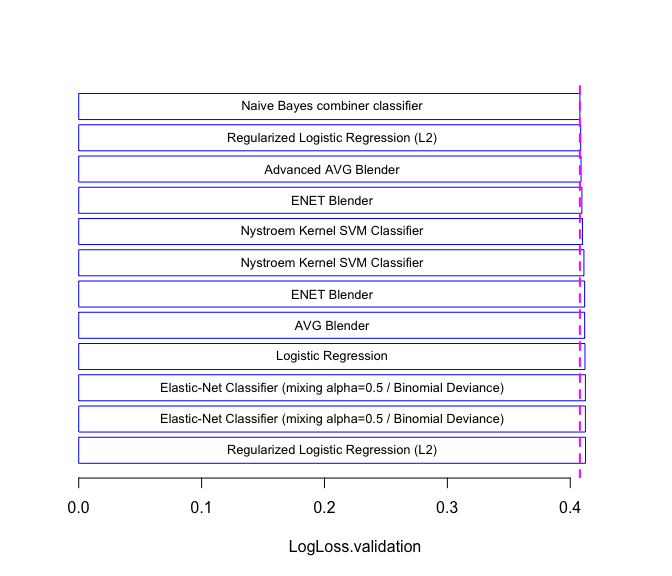Figure 7: Horizontal barplot LogLoss summary of the 64% models for the small loss data.

Figure 8 gives a summary of the area under the ROC curve (AUC) values for all models from the anomalous loss modeling project, color coded by the percentage of the training data used to fit each model. In particular, the models characterized in the barplot in Figure 7 are represented as red points in this plot, and it is clear that these models achieve AUC values between approximately 0.62 and 0.64. While these AUC values are large enough to suggest the loss anomaly is somewhat predictable from the other covariates, they are not large enough to suggest strong predictability, in contrast to the missing insulin data example.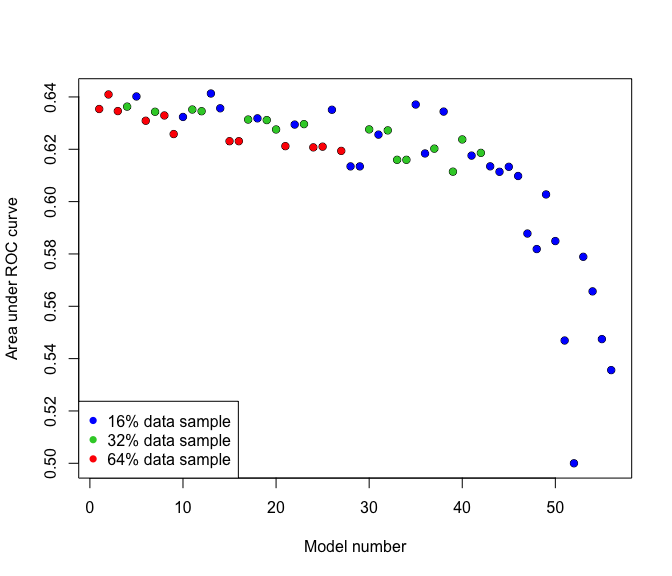Figure 8: Scatterplot summary of the AUC values for all models from AnomLossProject.

Despite the much lower predictability seen for this example, it is still of interest to ask which variables are most responsible for the differences that are seen here. We proceed as before, generating seven new DataRobot modeling projects, replacing each covariate in turn with its random permutation. Shifts in LogLoss and/or AUC values are then examined to see which variables appear most strongly related to the differences that are present between these data subsets. Figure 9 gives a beanplot summary of the shifts in AUC values seen in response to random permutations applied to each covariate, in the same general format as Figure 4 for the missing Pima Indians insulin data. Note, however, that since the best performance corresponds to the largest AUC value, downward shifts in this response variable are indicative of a worsening of model quality. Thus, the variable whose randomization worsens model quality the most, on average, is area, followed by veh_body, with agecat coming in as a distant third place. None of the other variables appear to be influential in predicting the anomalous $200 value for claimcst0. As before, it is important to note that some models exhibit unusual sensitivities to certain variables, much greater than the average. Here, a gradient boosted tree classifier exhibits the unusually large sensitivity to the veh_body value seen in Figure 9.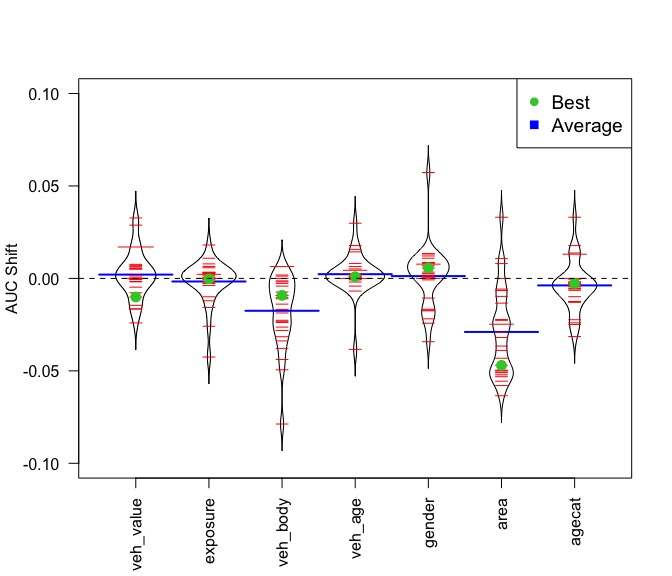Figure 9: Beanplot summary of AUC shifts versus random permutation. ## 4. Summary This note has presented two examples to illustrate how the problem of comparing two datasets can be converted into a binary classification problem, and how the datarobot R package can be usefully applied to this classification problem. The basic sequence is: 1. Merge the two datasets into a single composite dataset; 2. Define a binary response variable for the merged dataset indicating each record’s origin; 3. Fit a collection of binary classifiers of different types to predict this binary response; 4. Use binary classifier characterization measures like the area under the ROC curve to assess the degree of difference between the original datasets; 5. Use the random permutation-based variable importance measures described in Section 2.3 to identify variables associated with the observed differences between these datasets. The first example considered the question of whether the insulin variable in the Pima Indians diabetes data from the mlbench R package appeared to be missing randomly or systematically. It was seen that these missing insulin records were very strongly associated with the triceps skinfold thickness variable, and further examination revealed a very strong association between missing values in both variables. These results show that any missing data treatment strategy applied to this dataset needs to account for this association. Conversely, the results presented here provide no evidence of a systematic difference with respect to diabetic diagnosis between the missing insulin and non-missing insulin records. The second example considered an unusual subset of nonzero loss records in a publicly available vehicle insurance dataset, where the smallest nonzero loss is$200 exactly, and this value accounts for approximately 15% of all nonzero losses. Applying the strategy described here to compare this unusual record subset with the other nonzero loss records does suggest a difference, though less dramatic than in the first example. The variables most strongly associated with this difference are area, a 6-level categorical variable characterizing the geographic area of residence, veh_body, a 13-level categorical variable characterizing vehicle type, and to a smaller extent, agecat, an ordinal variable that characterizes driver age.

Finally, both examples illustrate the utility of basing our analysis on multiple models. In particular, the variable importance summaries presented in Figure 4 for the first example and Figure 9 for the second example both exhibit pronounced outliers, corresponding to models that are unusually sensitive to the absence of predictors that are not influential for the vast majority of the other models in the collection. By aggregating the results over all models in the collection, we can avoid excessive dependence on any individual model in the collection.